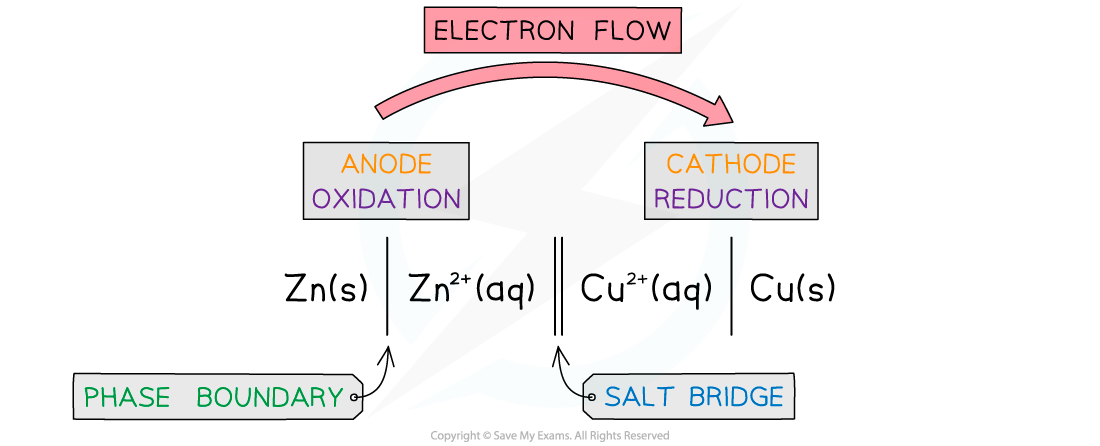# Edexcel A Level Chemistry:复习笔记6.1.4 Conventional Cell Representation

### Conventional Cell Representation

#### Conventional Representation of Cells

• As it is cumbersome and time-consuming to draw out every electrochemical cell in full, a system of notation is used which describes the cell in full, but does not require it to be drawn.
• An electrochemical cell can be represented in a shorthand way by a cell diagram (sometimes called cell representations or cell notations)The conventional representation of voltaic cells

• By convention, the half cell with the greatest negative potential is written on the left of the salt bridge, so Eθcell = Eθright – Eθleft
• In this case, Eθcell = +0.34 – -0.76 = +1.10 V.

• The left cell is being oxidized while the right is being reduced
• If there is more than one species in solution, and the species are on different sides of the half-equation, the different species are separated by a comma
• This method of representing electrochemical cells is known as the conventional representation of a cell, and it is widely used
• If both species in a half reaction are aqueous then an inert platinum electrode is needed which is recorded on the outside of the half cell diagram

#### Some Examples

• For the iron(II) and iron(III) half cell reaction a platinum electrode is needed as an electron carrier
• The half equation is

Fe3+(aq) + e- ⇌ Fe2+(aq)

• So the cell convention as a left hand electrode would be

Pt 丨Fe2+(aq), Fe3+(aq)

• Notice the order must be Fe(II) then Fe(III) as the left side is an oxidation reaction, so Fe(II) is oxidised to Fe(III) by the loss of an electron
• The platinum electrode is separated by the phase boundary (vertical solid line), but the iron(II) and iron(III) are separated by a comma since they are in the same phase
• Non-metals will also require a platinum electrode
• If chlorine is used as an electrode the reduction reaction is

Cl2(g) + 2e- ⇌ 2Cl-(aq)

• The conventional representation of the half reaction would be

Cl2 (g), 2Cl- (aq) | Pt

• Notice that the half cell reaction is balanced; however, it would be also correct to write it as

Cl2 (g), Cl- (aq) | Pt

• This is because conventional cell diagrams are not quantitative- they are just representations of the materials and redox processes going on
• Most chemists tend to show them balanced anyway

• Combining these two half cells together gives

Pt  | Fe2+(aq), Fe3+(aq)  ∥  Cl2 (g), 2Cl- (aq) | Pt

• As you can see the overall cell diagram is not quantitative as the left side is a one electron transfer and the right side is a two electron transfer